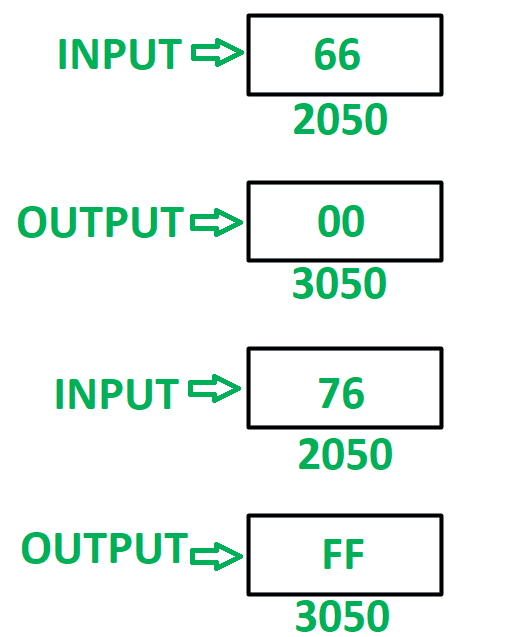# 8085 program to check whether both the nibbles of 8 bit number are equal or not

• Last Updated : 20 Jun, 2018

Problem – Write an assembly language program in 8085 microprocessor to check whether both the nibbles of 8 bit number are equal or not. If nibbles are equal then store 00 in memory location 3050 otherwise store FF in memory location 3050.

Example –Assumption – Number, to check for similar nibbles is stored at memory location 2050.

Algorithm –

1. Load the content of memory location 2050 in A.
2. Moves the content of A in B.
3. Mask the lower nibble and store it in register C.
4. Move the content of B in A.
5. Mask the higher order nibble and store it in A.
6. Reverse the content of A by using RLC instruction 4 times.
7. Compare the contents of A and C by help of CMP instruction. Update the flags of 8085.
8. Now store FF if ZF = 0 otherwise store 00 if ZF = 1.
9. Store the final result in memory location 3050.

Program –

2000 LDA 2050 A <- M
2003 MOV B, A B <- A
2004 ANI 0F A <- A (AND) 0F
2006 MOV C, A C <- A
2007 MOV A, B A <- B
2008 ANI F0 A <- A (AND) 0F
200A RLC Rotate content of A left by one bit without carry
200B RLC Rotate content of A left by one bit without carry
200C RLC Rotate content of A left by one bit without carry
200D RLC Rotate content of A left by one bit without carry
200E CMP C A – C
200F JZ 2018 Jump if ZF = 1
2013 MVI A, FF A <- FF
2018 MVI A, 00 A <- 00
201A STA 3050 M <- A
201D HLT END

Explanation – Registers A, B, C are used for general purpose.

1. LDA 2050: load the content of memory location 2050 in accumulator A.
2. MOV B, A: moves the content of A in register B.
3. ANI 0F: performs AND operation in contents of A and 0F. Store the result in A.
4. MOV C, A: moves the content of A in register C.
5. MOV A, B: moves the content of B in A.
6. ANI F0: performs AND operation in contents of A and F0. Store the result in A.
7. RLC: rotates content of A left by one bit without carry. Use the instruction 4 times to reverse the number.
8. CMP C: compares the content of A and C. Update the flags of 8085 accordingly.
9. JZ 2018: jump to memory location 2018 if zero flag is set.
10. MVI A, FF: assign FF to A.